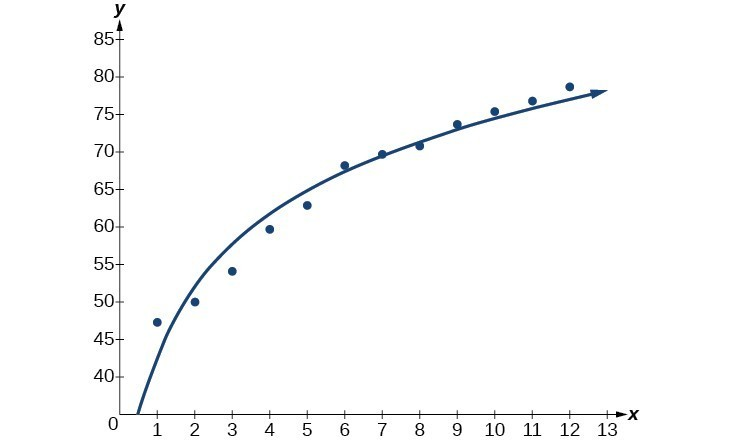## Build a logarithmic model from data

Just as with exponential functions, there are many real-world applications for logarithmic functions: intensity of sound, pH levels of solutions, yields of chemical reactions, production of goods, and growth of infants. As with exponential models, data modeled by logarithmic functions are either always increasing or always decreasing as time moves forward. Again, it is the way they increase or decrease that helps us determine whether a logarithmic model is best.

Recall that logarithmic functions increase or decrease rapidly at first, but then steadily slow as time moves on. By reflecting on the characteristics we’ve already learned about this function, we can better analyze real world situations that reflect this type of growth or decay. When performing logarithmic regression analysis, we use the form of the logarithmic function most commonly used on graphing utilities, $y=a+b\mathrm{ln}\left(x\right)$. For this function

• All input values, x, must be greater than zero.
• The point (1, a) is on the graph of the model.
• If b > 0, the model is increasing. Growth increases rapidly at first and then steadily slows over time.
• If < 0, the model is decreasing. Decay occurs rapidly at first and then steadily slows over time.

### A General Note: Logarithmic Regression

Logarithmic regression is used to model situations where growth or decay accelerates rapidly at first and then slows over time. We use the command “LnReg” on a graphing utility to fit a logarithmic function to a set of data points. This returns an equation of the form,

$y=a+b\mathrm{ln}\left(x\right)$

Note that

• all input values, x, must be non-negative.
• when > 0, the model is increasing.
• when < 0, the model is decreasing.

### How To: Given a set of data, perform logarithmic regression using a graphing utility.

1. Use the STAT then EDIT menu to enter given data.
1. Clear any existing data from the lists.
2. List the input values in the L1 column.
3. List the output values in the L2 column.
2. Graph and observe a scatter plot of the data using the STATPLOT feature.
1. Use ZOOM  to adjust axes to fit the data.
2. Verify the data follow a logarithmic pattern.
3. Find the equation that models the data.
1. Select “LnReg” from the STAT then CALC menu.
2. Use the values returned for a and b to record the model, $y=a+b\mathrm{ln}\left(x\right)$.
4. Graph the model in the same window as the scatterplot to verify it is a good fit for the data.

### Example 2: Using Logarithmic Regression to Fit a Model to Data

Due to advances in medicine and higher standards of living, life expectancy has been increasing in most developed countries since the beginning of the 20th century.

The table below shows the average life expectancies, in years, of Americans from 1900–2010.

 Year 1900 1910 1920 1930 1940 1950 Life Expectancy(Years) 47.3 50 54.1 59.7 62.9 68.2 Year 1960 1970 1980 1990 2000 2010 Life Expectancy(Years) 69.7 70.8 73.7 75.4 76.8 78.7
1. Let x represent time in decades starting with = 1 for the year 1900, = 2 for the year 1910, and so on. Let y represent the corresponding life expectancy. Use logarithmic regression to fit a model to these data.
2. Use the model to predict the average American life expectancy for the year 2030.

### Solution

1. Using the STAT then EDIT menu on a graphing utility, list the years using values 1–12 in L1 and the corresponding life expectancy in L2. Then use the STATPLOT feature to verify that the scatterplot follows a logarithmic pattern.

Use the “LnReg” command from the STAT then CALC menu to obtain the logarithmic model,

$y=42.52722583+13.85752327\mathrm{ln}\left(x\right)$Figure 4

Next, graph the model in the same window as the scatterplot to verify it is a good fit.

2. To predict the life expectancy of an American in the year 2030, substitute = 14 for the in the model and solve for y:
$\begin{cases}y\hfill & =42.52722583+13.85752327\mathrm{ln}\left(x\right)\hfill & \text{Use the regression model found in part (a)}\text{.}\hfill \\ \hfill & =42.52722583+13.85752327\mathrm{ln}\left(14\right)\hfill & \text{Substitute 14 for }x\text{.}\hfill \\ \hfill & \approx \text{79}\text{.1}\hfill & \text{Round to the nearest tenth.}\hfill \end{cases}$

If life expectancy continues to increase at this pace, the average life expectancy of an American will be 79.1 by the year 2030.

### Try It 2

Sales of a video game released in the year 2000 took off at first, but then steadily slowed as time moved on. The table below shows the number of games sold, in thousands, from the years 2000–2010.

 Year 2000 2001 2002 2003 2004 2005 Number Sold (thousands) 142 149 154 155 159 161 Year 2006 2007 2008 2009 2010 — Number Sold (thousands) 163 164 164 166 167 —

a. Let x represent time in years starting with = 1 for the year 2000. Let y represent the number of games sold in thousands. Use logarithmic regression to fit a model to these data.
b. If games continue to sell at this rate, how many games will sell in 2015? Round to the nearest thousand.

Solution

1. Source: Center for Disease Control and Prevention, 2013